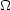### 8. CONSTRAINTS ON COSMOLOGIES

The most important outcome of the newer experimental results is that the standard cosmological paradigm is in good shape. A large amount of high precision data on the power spectrum is adequately fit with fewer than 10 free parameters. The framework is that of Friedmann-Robertson-Walker models, which have nearly flat geometry, containing Dark Matter and Dark Energy, and with adiabatic perturbations having close to scale invariant initial conditions.

Within this framework, bounds can be placed on the values of the cosmological parameters. Of course, much more stringent constraints can be placed on models which cover a restricted number of parameters, e.g. assuming thattot = 1, n = 1 or r = 0. More generally, the constraints depend upon the adopted priors, even if they are implicit, for example by restricting the parameter freedom or the ranges of parameters (particularly where likelihoods peak near the boundaries), or by using different choices of other data in combination with the CMB. When the data become even more precise, these considerations will become less important, but for now we caution that restrictions on model space and choice of priors need to be kept in mind when adopting specific parameter values and uncertainties.

There are some combinations of parameters that fit the CMB anisotropies almost equivalently. For example, there is a nearly exact geometric degeneracy, where any combination ofM andthat gives the same angular diameter distance to last scattering will give nearly identical Cs. There are also other near degeneracies among the parameters. Such degeneracies can be broken when using the CMB data in combination with other cosmological data sets. Particularly useful are complementary constraints from galaxy clustering, the abundance of galaxy clusters, weak gravitational lensing measurements, Type Ia supernova distances and the distribution of Lymanforest clouds. For an overview of some of these other cosmological constraints, see Ref. .

The combination of WMAP, CBI and ACBAR, together with weak priors (on h andB h2 for example), and within the context of a 6 parameter family of models (which fixestot = 1), yields the following results : A = 2.7( ± 0.3) × 10-9, n = 0.97 ± 0.03, h = 0.73 ± 0.05,B h2 = 0.023 ± 0.001,M h2 = 0.13 ± 0.01 and= 0.17 ± 0.07. Note that for h, the CMB data alone provide only a very weak constraint, unless spatial flatness or some other cosmological data are used. ForB h2 the precise value depends sensitively on how much freedom is allowed in the shape of the primordial power spectrum (see `Big-Bang Nucleosynthesis' mini-review ). For the optical depth, the error bar is large enough that apparently quite different results can come from other combinations of data.

The best constraint ontot is 1.02 ± 0.02. This comes from including priors from h and supernova data. Slightly different, but consistent results come from using different data combinations.

The 95% confidence upper limit on r is 0.53 (including some extra constraint from galaxy clustering). This limit is stronger if we restrict ourselves to n < 1 and weaker if we allow dn / d ln k0.

There are also constraints on parameters over and above the basic 8 that we have described. But for such constraints it is necessary to include additional data in order to break the degeneracies. For example the addition of the Dark Energy equation of state, w adds the partial degeneracy of being able to fit a ridge in (w, h) space, extending to low values of both parameters. This degeneracy is broken when the CMB is used in combination with independent H0 limits, for example , giving w < - 0.5 at 95% confidence. Tighter limits can be placed using restrictive model-spaces and/or additional data.

For the optical depth, the error bar is large enough that apparently quite different results can come from other combinations of data. The constraint from the combined WMAP CTT and CTE data is= 0.17 ± 0.04, which corresponds (within reasonable models) to a reionization redshift 9 < zi < 30 (95% CL) . This is a little higher than some theoretical predictions and some suggestions from studies of absorption in high-z quasar spectra . The excitement here is that we have direct information from CMB polarization which can be combined with other astrophysical measurements to understand when the first stars formed and brought about the end of the cosmic dark ages.